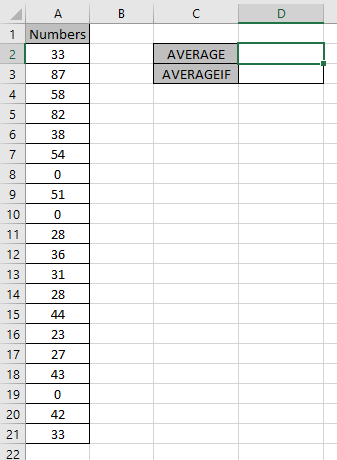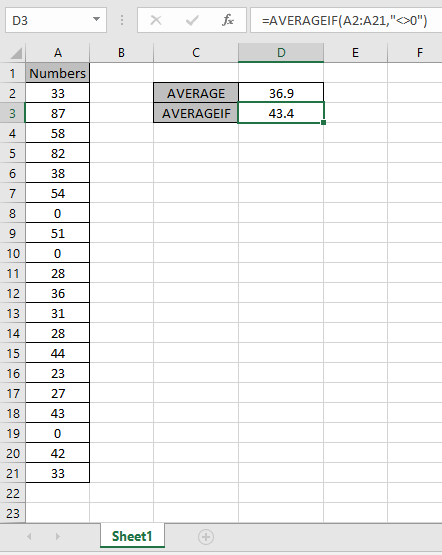# How to Ignore zero in the Average of numbers in Excel

In this article, we will learn about how to get the Average of numbers ignoring zero in Excel.

In simple words, Average of numbers is the ratio of sum of the numbers to the total number of values in the data.

Average  =  Sum of values ( numbers ) / number of values ( numbers )

AVERAGEIF function returns the average of the numbers which satisfies the condition. Syntax:

=AVERAGEIF(range,"<>0")

Range : numbers or values

<>0 : condition, matches values that are not equal to zero.

Let’s get this function using it in an example.

Here we have a list of numbers from A2:A21.Firstly, we will calculate Average of the numbers considering 0s.As you can see in the sanpshot above the AVERAGE of number using the AVERAGE function.

The below mentioned formula will accept values except 0. Use the formula:

=AVERAGEIF(A2:A21, "<>0")

A2:A21 : range “<>0” : condition for ignoring zero values.As you can see the difference in the values. The Average of the numbers ignoring zero is 43.4.
NOTE: For Blank cells One more thing that is to understand is the AVERAGEAVERAGEIF & AVERAGEIFS function ignores blank cells (and cells that contain text values). So there is no need to filter results.

Hope you understood how to get the Average of numbers ignoring zero in Excel. Explore more articles on Excel mathematical functions here. Please feel free to state your query or feedback for the above article.

Related Articles

How to get the Average Difference between lists in Excel

How to Calculate Weighted Average in Excel

Ignore zero in the Average of numbers in Excel

How to use the AVERAGEIF function in excel

How to use the AVERAGEIFS function in excel

Popular Articles

Edit a dropdown list

If with conditional formatting

If with wildcards

Vlookup by date

Terms and Conditions of use

The applications/code on this site are distributed as is and without warranties or liability. In no event shall the owner of the copyrights, or the authors of the applications/code be liable for any loss of profit, any problems or any damage resulting from the use or evaluation of the applications/code.